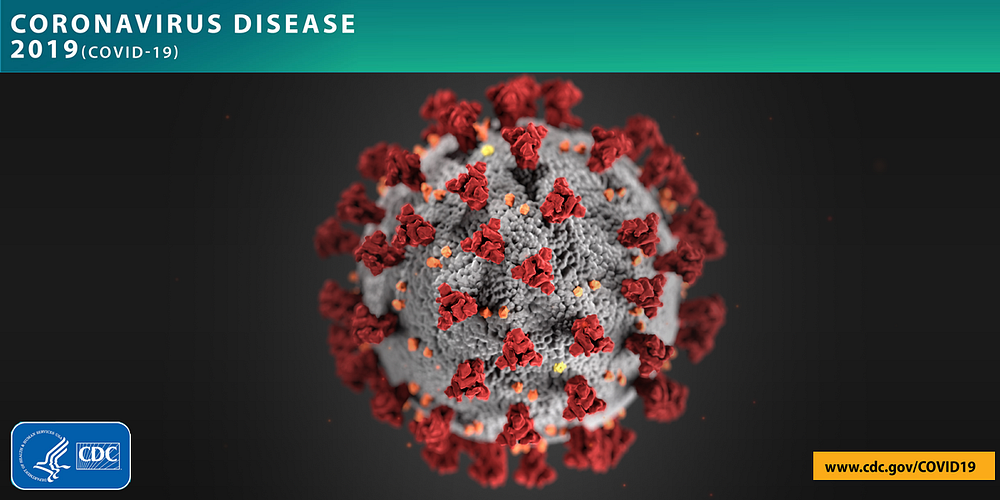# Detecting COVID-19 using X-ray images with the Convolutional Neural Network

Original article can be found here (source): Deep Learning on Medium# Detecting COVID-19 using X-ray images with the Convolutional Neural Network

In this tutorial i will show you the result of applying SqueezeNet  on X-ray images for detecting the new Corona virus (2019).

The CoVid-19 (Coronavirus disease 2019) is a new generation of the Corona infectious virus which started in Wuhan, China and become a pandemic in 2020 and spread in all over the world .

According to a new study done by Wang, et. al in China, the analysis of Computerized Tomography (CT) using Deep Learning can be faster than the usual test with good results. The researcher in this study applied convolutional neural network ( Inception network) on 1,119 CT scans.

So, In this tutorial I implemented a model for COVID-19 detection using X-ray scans, the models are trained using the SqueezeNet architecture which is a lightweight architecture of CNN based on 50 images, which 25 images are normal cases and 25 images are positive cases of the COVID-19.

The dataset is very limited, so we applied the transfer learning techniques and the data augmentation techniques.

So, let’s start coding.

Firstly, we import all the libraries and the functions we need.

import numpy
from keras.applications.imagenet_utils import preprocess_input
from keras.models import Model
from keras_squeezenet import SqueezeNet
from keras.layers import GlobalAveragePooling2D, Dense
from keras.preprocessing.image import ImageDataGenerator
from keras.callbacks import EarlyStopping, ModelCheckpoint, ReduceLROnPlateau
from keras import optimizers

Secondly, we initialise our Hyper-parameters

NUM_CLASSES = 2 #number of classes (normal,covid)
CHANNELS = 3 #number of images channels
image_size = 224 #input image size
DENSE_LAYER_ACTIVATION = ‘softmax’ #activation function of last layer
OBJECTIVE_FUNCTION = ‘binary_crossentropy’ #Loss function

LOSS_METRICS = [‘accuracy’] #loss metrics
NUM_EPOCHS = 100 #number of epochs
EARLY_STOP_PATIENCE = 30 #the number epochs before the early stopping

BATCH_SIZE_TRAINING = 16 #the training batch size
BATCH_SIZE_VALIDATION = 16 #the validation batch size

Thirdly, model creation:

base_model = SqueezeNet(include_top = False, weights = ‘imagenet’, input_shape = [image_size,image_size,3])
x = base_model.output
x = GlobalAveragePooling2D()(x)
preds = Dense(NUM_CLASSES,activation=DENSE_LAYER_ACTIVATION)(x)
model = Model(inputs=base_model.input,outputs=preds)

model.compile(optimizer = opt, loss = OBJECTIVE_FUNCTION, metrics = LOSS_METRICS)

Fourthly, the data generation:

data_generator = ImageDataGenerator(rescale=1.0/255.0,
preprocessing_function=preprocess_input,
horizontal_flip=True,
vertical_flip=True,
zoom_range=0.01,
shear_range=0.2,
brightness_range=[0.2,1.0])

data_generator2 = ImageDataGenerator(rescale=1.0/255.0,preprocessing_function=preprocess_input)

train_generator = data_generator.flow_from_directory(
‘trainset/’,
target_size=(image_size, image_size),
batch_size=BATCH_SIZE_TRAINING,
class_mode=’binary’)

validation_generator = data_generator2.flow_from_directory(
‘testset/’,
target_size=(image_size, image_size),
batch_size=BATCH_SIZE_VALIDATION,
class_mode=’binary’)

Finally, the model training:

fit_history = model.fit_generator(train_generator,
epochs = NUM_EPOCHS,
validation_data=validation_generator,
verbose=2,
callbacks = [cb_checkpointer, cb_early_stopper, reduce_lr])

The full code and the dataset are in a github repository here.